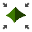# Scale Wedge

The Scale Wedge option allows you to scale the size of wedges according to Joint Trace Length, Joint Persistence, Wedge Volume or Weight.

To use the Scale Wedge option:

1. Select Scale Wedgefrom the Analysis menu.
2. The current wedge parameters (e.g. Trace Length, Persistence, Volume) are listed in the dialog.
3. Select the check box(es) for the desired scaling parameter(s) and enter a Scaling Value for each selected parameter. See the note below about using multiple scaling parameters.
4. Select Apply or OK, and the analysis will be re-run, using the scaled wedge size. All analysis results will now apply to the scaled wedge size.

Further information about the Scaling options is given below.

## Trace Length

Wedge size can be scaled according to the Trace Length of Joint 1 or Joint 2. The Trace Lengths may occur in EITHER the Slope Face or the Upper Face. For a given joint the maximum of the Slope Face or Upper Face trace length is used.

For example, if the Trace Length of Joint 1 is 10 meters on the Upper Face, and 15 meters on the Lower Face, then the current value of Joint 1 Trace Length will be 15 meters, and the scaling will be applied to the Trace Length of Joint 1 on the Slope Face.

The Trace Lengths for Joint 1 and Joint 2 on both the Slope Face and Upper Face, are listed in the Info Viewer.

## Maximum Persistence

The Maximum Persistence, as defined in SWedge, refers to the maximum dimension between any two points, as measured on the wedge failure surfaces (Joint 1 and Joint 2).

## Wedge Volume/Weight

Wedge size can be scaled according to Wedge Volume or Wedge Weight. Note that the wedge volume and weight are directly related to the rock unit weight (i.e. wedge weight = wedge volume * rock unit weight).

## Multiple Scaling Values

If multiple scaling parameters are entered in the Scale Wedge dialog, only the scaling parameter which gives the smallest wedge will be applied, for a given wedge. All other scaling values will be ignored.

## Probabilistic/Combination Analysis and Scaling

For a Probabilistic or Combination Analysis, multiple scaling parameters can be useful if the wedge plane orientations are random variables, because different scaling parameters may be applied to different wedges. However, for any individual wedge generated by a Probabilistic or Combination analysis, only the scaling parameter which gives the smallest wedge will be applied.

The Scale Wedge option cannot be used in conjunction with the Persistence Analysis option. If the Persistence Analysis option is in use, then the Scale Wedge option will NOT be available, and vice-versa.